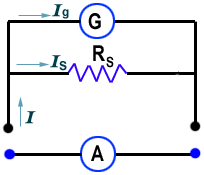Physics

# How to Measure the Unit of Electric Current?

Unit: The unit of electric current is ampere. If an amount of charge 1 C flows in 1 second through any cross-section of a conductor, then the quantity of electric current produced is called 1 A.

I = 1 C / 1s = 1 Cs1

= 1 A

In an isolated charged conductor, the charge stays on its surface and do not move. Such type of charges is called an electrostatic charge. However, if we can provide a conducting path, the charges will flow instead of being bound on the conductor. When it happens, we say an electric current is produced.How electric current is produced from moving charges is described in terms of the circuit as shown in the figure. At the start of the experiment, two plug keys Ig and are taken out and the two metal plates A and B are uncharged by touching with hand. Now, if the plug Ig is closed, the high voltage source will be connected to the two metal plates.

Next, switch on the high voltage source to charge up the two metal plates positively and negatively by an equal amount. Now, key Ig is removed and key Is is plugged in to provide a continuous conducting path linking the positively and negatively charged metal plates to the galvanometer. Here, the galvanometer is a device that can detect the existence of the flow of current. It would be observed that the pointer in the galvanometer is seen to deflect momentarily to one side and then quickly return to its initial position.

The galvanometer’s deflection shows that an electric current is produced. How is this electric current produced? The current is caused by the flow of electrons from the negatively charged plate B through the galvanometer and then to positively charged plate A. The positive charges of plate A are neutralized by the incoming negatively charged electrons. As a result, the transient current which is detected by the galvanometer is produced due to the discharge of the two metal plates.